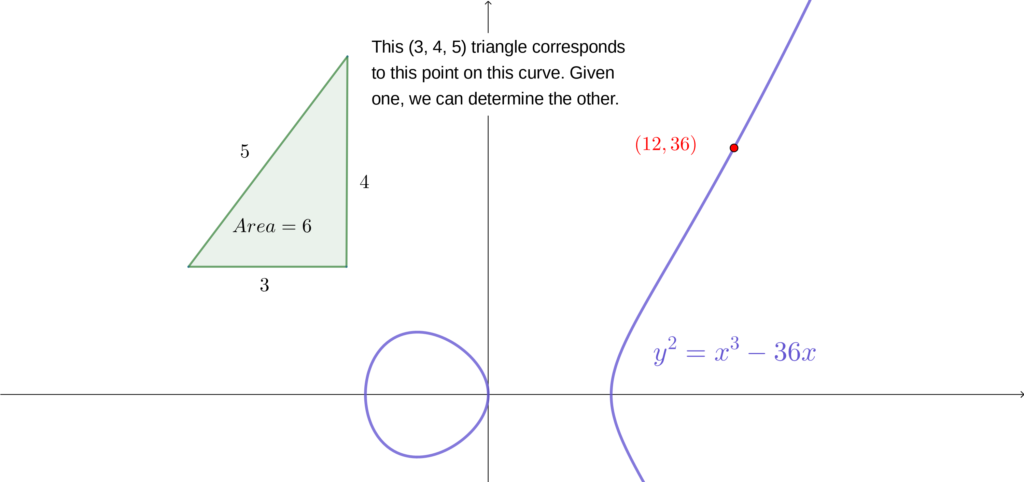# Talk: Finding Congruent Numbers, Arithmetic Progressions of Squares, and Triangles

Here are some notes for my talk Finding Congruent Numbers, Arithmetic Progressions of Squares, and Triangles (an invitation to analytic number theory), which I’m giving on Tuesday 26 February at Macalester College.The slides for my talk are available here.

The overarching idea of the talk is to explore the deep relationship between

1. right triangles with rational side lengths and area $n$,
2. three-term arithmetic progressions of squares with common difference $n$, and
3. rational points on the elliptic curve $Y^2 = X^3 – n^2 X$.

If one of these exist, then all three exist, and in fact there are one-to-one correspondences between each of them. Such an $n$ is called a congruent number.

By understanding this relationship, we also describe the ideas and results in the paper A Shifted Sum for the Congruent Number Problem, which I wrote jointly with Tom Hulse, Chan Ieong Kuan, and Alex Walker.

Towards the end of the talk, I say that in practice, the best way to decide if a (reasonably sized) number is congruent is through elliptic curves. Given a computer, we can investigate whether the number $n$ is congruent through a computer algebra system like sage.1

For the rest of this note, I’ll describe how one can use sage to determine whether a number is congruent, and how to use sage to add points on elliptic curves to generate more triangles corresponding to a particular congruent number.

Firstly, one needs access to sage. It’s free to install, but it’s quite large. The easiest way to begin using sage immediately is to use cocalc.com,  a free interface to sage (and other tools) that was created by William Stein, who also created sage.

In a sage session, we can create an elliptic curve through


> E6 = EllipticCurve([-36, 0])
> E6
Elliptic Curve defined by y^2 = x^3 - 36*x over Rational Field


More generally, to create the curve corresponding to whether or not $n$ is congruent, you can use


> n = 6   # (or anything you want)
> E = EllipticCurve([-n**2, 0])


We can ask sage whether our curve has many rational points by asking it to (try to) compute the rank.


> E6.rank()
1


If the rank is at least $1$, then there are infinitely many rational points on the curve and $n$ is a congruent number. If the rank is $0$, then $n$ is not congruent.2

For the curve $Y^2 = X^3 – 36 X$ corresponding to whether $6$ is congruent, sage returns that the rank is $1$. We can ask sage to try to find a rational point on the elliptic curve through


> E6.point_search(10)
[(-3 : 9 : 1)]


The 10 in this code is a limit on the complexity of the point. The precise definition isn’t important — using $10$ is a reasonable limit for us.

We see that this output something. When sage examines the elliptic curve, it uses the equation $Y^2 Z = X^3 – 36 X Z^2$ — it turns out that in many cases, it’s easier to perform computations when every term is a polynomial of the same degree. The coordinates it’s giving us are of the form $(X : Y : Z)$, which looks a bit odd. We can ask sage to return just the XY coordinates as well.


> Pt = E6.point_search(10)  # The  means to return the first element of the list
> Pt.xy()
(-3, 9)


In my talk, I describe a correspondence between points on elliptic curves and rational right triangles. In the talk, it arises as the choice of coordinates. But what matters for us right now is that the correspondence taking a point $(x, y)$ on an elliptic curve to a triangle $(a, b, c)$ is given by
$$(x, y) \mapsto \Big( \frac{n^2-x^2}{y}, \frac{-2 \cdot x \cdot y}{y}, \frac{n^2 + x^2}{y} \Big).$$

We can write a sage function to perform this map for us, through


> def pt_to_triangle(P):
x, y = P.xy()
return (36 - x**2)/y, (-2*x*6/y), (36+x**2)/y

> pt_to_triangle(Pt)
(3, 4, 5)


This returns the $(3, 4, 5)$ triangle!

Of course, we knew this triangle the whole time. But we can use sage to get more points. A very cool fact is that rational points on elliptic curves form a group under a sort of addition — we can add points on elliptic curves together and get more rational points. Sage is very happy to perform this addition for us, and then to see what triangle results.


> Pt2 = Pt + Pt
> Pt2.xy()
(25/4, -35/8)
> pt_to_triangle(Pt2)
(7/10, 120/7, -1201/70)


Another rational triangle with area $6$ is the $(7/10, 120/7, 1201/70)$ triangle. (You might notice that sage returned a negative hypotenuse, but it’s the absolute values that matter for the area). After scaling this to an integer triangle, we get the integer right triangle $(49, 1200, 1201)$ (and we can check that the squarefree part of the area is $6$).

Let’s do one more.


> Pt3 = Pt + Pt + Pt
> Pt3.xy()
(-1587/1369, -321057/50653)
> pt_to_triangle(Pt3)
(-4653/851, -3404/1551, -7776485/1319901)


That’s a complicated triangle! It may be fun to experiment some more — the triangles rapidly become very, very complicated. In fact, it was very important to the main result of our paper that these triangles become so complicated so quickly!

#### Footnotes

1. There are other computer algebra systems with elliptic curve functionality, but sage is free and open-source, and I help contribute to and develop sage so I’m biased.
2. Behind the scenes, sage is using a particular C++ library written by John Cremona (who happens to have been my postdoctoral advisor) to try to compute the rank of the curve. This usually works, but for large inputs it can sometimes time out or fail. This approach has limitations.
This entry was posted in Expository, Math.NT, Mathematics, Programming, sage, sagemath, sagemath. Bookmark the permalink.